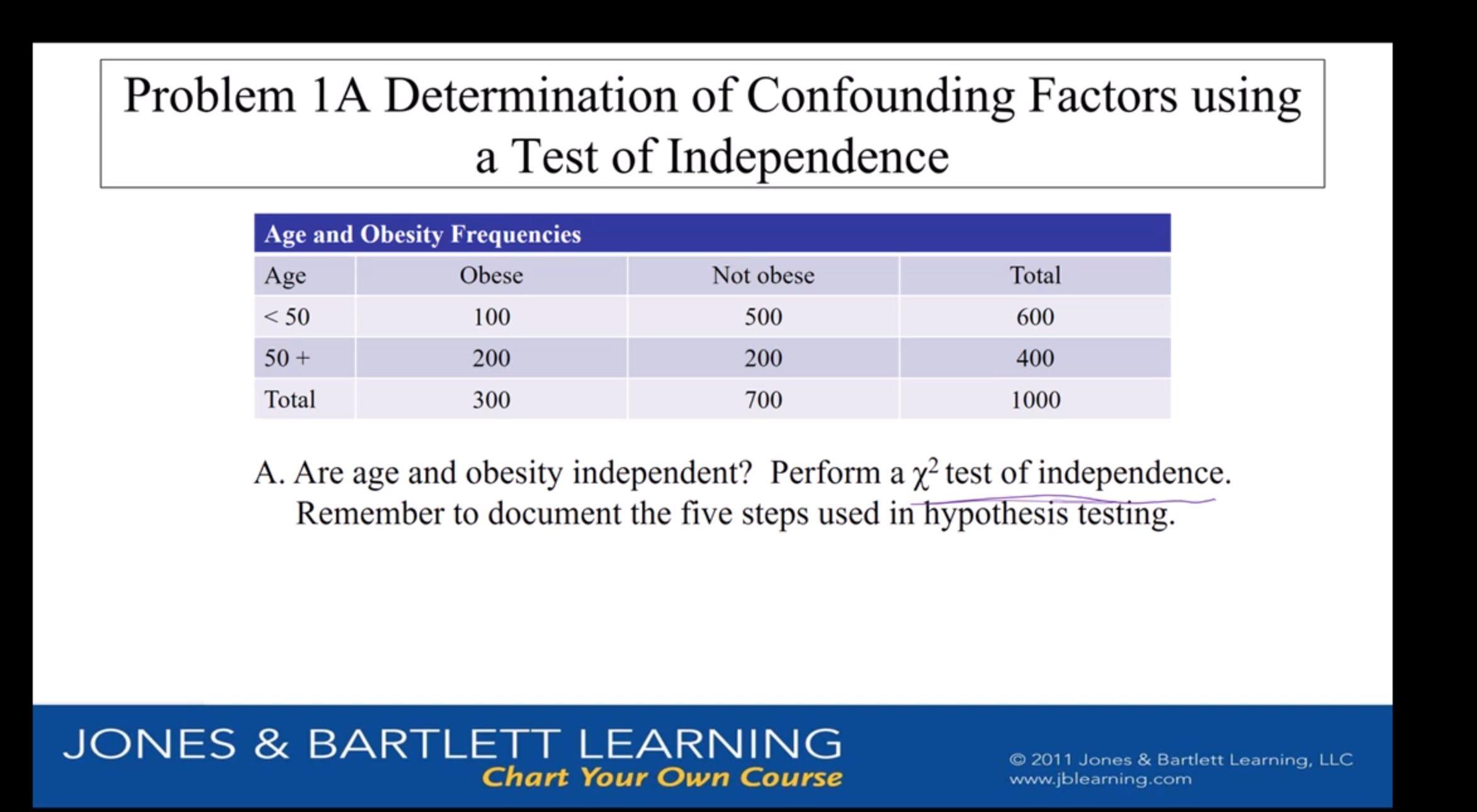# Problem 1A Determination of Confounding Factors usinga Test of IndependenceAge and Obesity FrequenciesObeseNot obeseTotalAge500100600

Question
96 viewshelp_outlineImage TranscriptioncloseProblem 1A Determination of Confounding Factors using a Test of Independence Age and Obesity Frequencies Obese Not obese Total Age 500 100 600 <50 50+ 200 200 400 Total 1000 300 700 A. Are age and obesity independent? Perform a y2 test of independence. Remember to document the five steps used in hypothesis testing. JONES& BARTLETT LEARNING Chart Your Own Course O2011 Jones & Bartlett Learning, LLC www.jblearning.com fullscreen
check_circle

Step 1

Let us first test whether the “age” and “obesity” are independent or not, using a Chi-square test of Independence. If the two variables are found not to be independent, then, by observing the change in the proportions, it can be decided whether the age of an individual is independent of obesity.

Step 2

Hypotheses:

The null hypothesis is:

H : The age and obesity are not attribute differently.

The alternative hypothesis is:

H : The age and obesity are attribute differently.

Step 3

Calculation steps:

The calculations have been done in EXCEL.

Denote oij as the observed frequency for ith row and jth column (i =1, 2; j =1, 2) and eij as the expected frequency for ith row and jth column.

Table 1 provides the data values, oij.

Table 2 calculates the row proportions: (cell value)/(row total) = oij / oi∙ so that, the first row proportion will be 100/600 ≈ 0.1667. Similarly, the others can be calculated.

Table 3 calculates the expected frequencies under the independence assumption. If independent, ...

### Want to see the full answer?

See Solution

#### Want to see this answer and more?

Solutions are written by subject experts who are available 24/7. Questions are typically answered within 1 hour.*

See Solution
*Response times may vary by subject and question.
Tagged in

### Hypothesis Testing